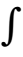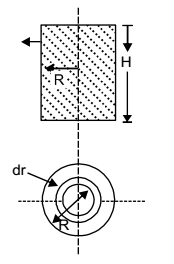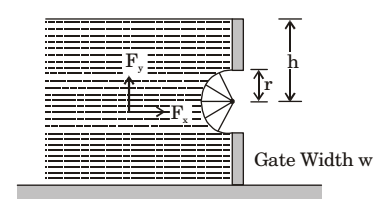## Fluid Mechanics and Hydraulic Machinery Miscellaneous

#### Fluid Mechanics and Hydraulic Machinery

1. Which combination of the following statements about steady incompressible forced vortex flow is correct?
P: Shear stress is zero at all points in the flow.
Q: Vorticity is zero at all points in the flow.
R: Velocity is directly proportional to the radius from the centre of the vortex.
S: Total mechanical energy per unit mass is constant in the entire flow field.
Select the correct answer using the codes given below:

1. Clearly zero shear stress and vortex.

##### Correct Option: B

Clearly zero shear stress and vortex.

1. The 2-D flow with, velocity
V̄ = (x + 2y + 2) î + (4 – y) ĵ is

1. v̄ = (x + 2y +2)î + (4 - y)ĵ
u =x + 2y + 2, v = 4 – y

 ∴ δv = 1, δv = - 1 δx δy

 ∴ δv + 1, δv = 0 δx δy

hence in compressible.
 Again, ω = 1δv - δv2 δx δy

 = 1 (0 - 2) = - 1. 2

hence not irrotational.

##### Correct Option: D

v̄ = (x + 2y +2)î + (4 - y)ĵ
u =x + 2y + 2, v = 4 – y

 ∴ δv = 1, δv = - 1 δx δy

 ∴ δv + 1, δv = 0 δx δy

hence in compressible.
 Again, ω = 1δv - δv2 δx δy

 = 1 (0 - 2) = - 1. 2

hence not irrotational.

1. Existence of velocity potential implies that

1. Fluid is irrotational

##### Correct Option: B

Fluid is irrotational

1. A closed cylinder having a radius R and height H is filled with oil of density ρ. If the cylinder is rotated about its axis at an angular velocity of ω, the thrust at the bottom of the cylinder is

1. We know that

 δp = ρv2 = ρ.ω2r2 = δω2r δr r r

[∵ v = ω × r]p δp =r ρω2r dr 0 0

 p = ρ ω2r2 2

Area of circular ring = 2πrdr
Force on elementry ring
= Intensity of pressure × Area of ring
 = ρ ω2r22πr dr 2

∴ Total force on the top of the cylinder=R ρ ρω2r22π r dr 0 2

 = ρ ρω2r22πR r3 dr 2 0

 ρ . ω22π R2 = ρ ω2 × πR4 2 4 4

Thrust at the bottom of the cylinder
= Weight of water in cylinder + Total force on the top of cylinder
 = ρg × πR2 × H + ρ ω2 × πR4 4

 = πR2ρω2R2 + ρgH4

##### Correct Option: D

We know that

 δp = ρv2 = ρ.ω2r2 = δω2r δr r r

[∵ v = ω × r]p δp =r ρω2r dr 0 0

 p = ρ ω2r2 2

Area of circular ring = 2πrdr
Force on elementry ring
= Intensity of pressure × Area of ring
 = ρ ω2r22πr dr 2

∴ Total force on the top of the cylinder=R ρ ρω2r22π r dr 0 2

 = ρ ρω2r22πR r3 dr 2 0

 ρ . ω22π R2 = ρ ω2 × πR4 2 4 4

Thrust at the bottom of the cylinder
= Weight of water in cylinder + Total force on the top of cylinder
 = ρg × πR2 × H + ρ ω2 × πR4 4

 = πR2ρω2R2 + ρgH4

1. The horizontal and vertical hydro static forces Fx and Fy on the semicircular gate, having a width w into the plane of figure, are1. Fx = 2ρghrw

 Fy = w2 - w1 πr2 . ρg × w = πr2ρgw 2 2

##### Correct Option: D

Fx = 2ρghrw

 Fy = w2 - w1 πr2 . ρg × w = πr2ρgw 2 2